Question

# The demand curve and supply curve for​ one-year discount bonds with a face value of ​\$1,050...

The demand curve and supply curve for​ one-year discount bonds with a face value of ​\$1,050 are represented by the following​ equations:

Bd​: Price equals negative 0.8 Quantity plus1,160 Bs​:

Price equals Quantity plus 680

The expected equilibrium quantity of bonds is nothing. ​(Round your response to the nearest whole​ number.)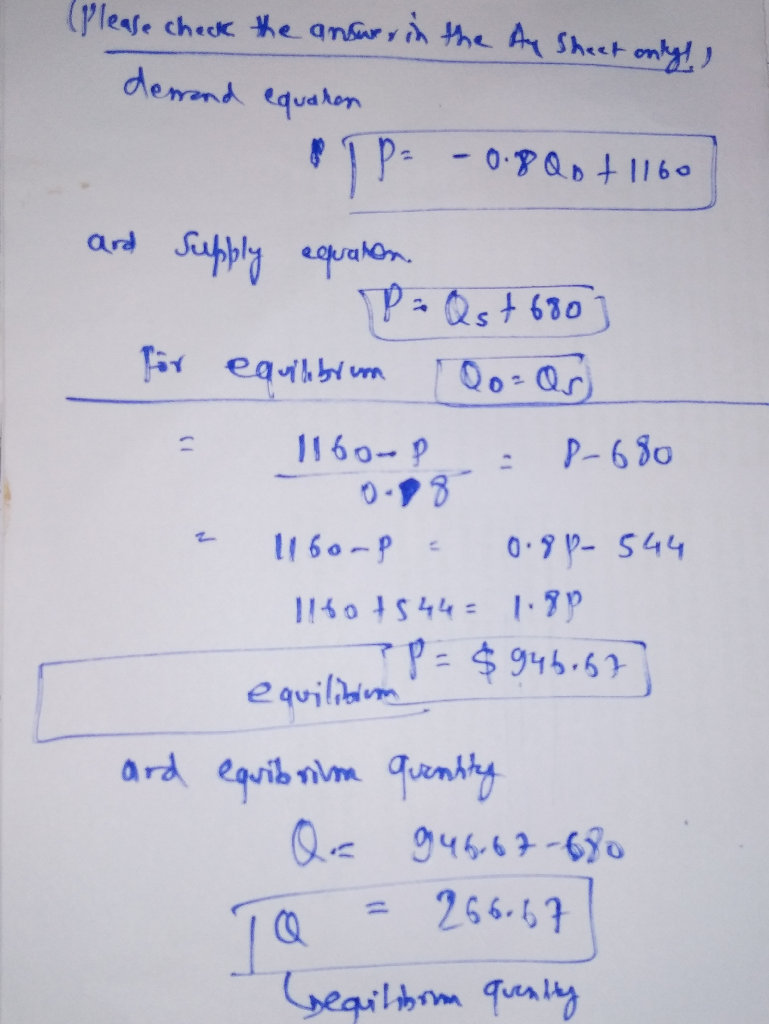#### Earn Coins

Coins can be redeemed for fabulous gifts.

Similar Homework Help Questions
• ### demand and supply curve for one-year discount bonds with face value of S100 are represented by...

demand and supply curve for one-year discount bonds with face value of S100 are represented by the following equation: Demand for bonds: Price ＝-0.8*Quantity+ 1,160 Supply of bonds: Price= Quantity +720 What is the expected equilibrium price? (2%) What is the expected interest rate in this market? (2%)

• ### Suppose that the demand and supply curves for one-year discount bonds with a face value of...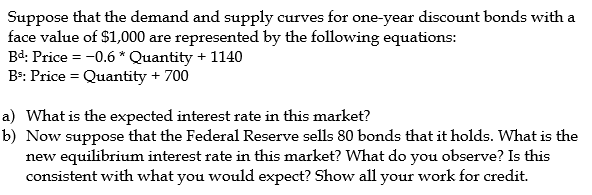Suppose that the demand and supply curves for one-year discount bonds with a face value of \$1,000 are represented by the following equations: Bd: Price = -0.6* Quantity + 1140 BS: Price = Quantity + 700 a) What is the expected interest rate in this market? b) Now suppose that the Federal Reserve sells 80 bonds that it holds. What is the new equilibrium interest rate in this market? What do you observe? Is this consistent with what you would...

• ### The demand curve and Supply Curve for one year discount bond with a face value of...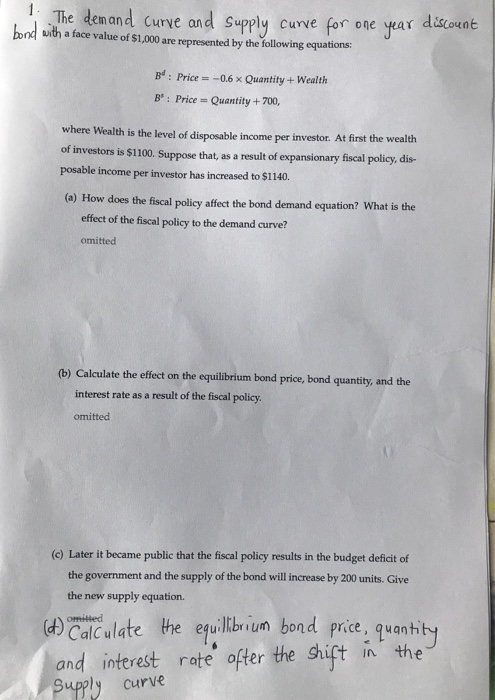The demand curve and Supply Curve for one year discount bond with a face value of \$1,000 are represented by the following equations B": Price = -0.6 x Quantity + Wealth B': Price = Quantity + 700, Where Wealth is the level of disposable income per investor. At first the wealth of investors is \$1100. Suppose that, as a result of expansionary fiscal policy, dis- posable income per investor has increased to \$1140. (a) How does the fiscal policy affect...

• ### i. Consider the following demand and supply equations for one-year discount bonds with a face value of \$1000. Bd: P = -0.6Q+1140 Bs: P = Q + 700 (6pts) Solve for the equilibrium values of P* and...

i. Consider the following demand and supply equations for one-year discount bonds with a face value of \$1000. Bd: P = -0.6Q+1140 Bs: P = Q + 700 (6pts) Solve for the equilibrium values of P* and Q*, as well as i*. 6pts. (8pts) Suppose that, as a result of a monetary policy action, the Fed sells 80 bonds that it holds. Assume that Bd and Md are held constant. Write down the new BS equation. Calculate the effect of...

• ### I need help figuring out the second part of the question. The demand curve and supply...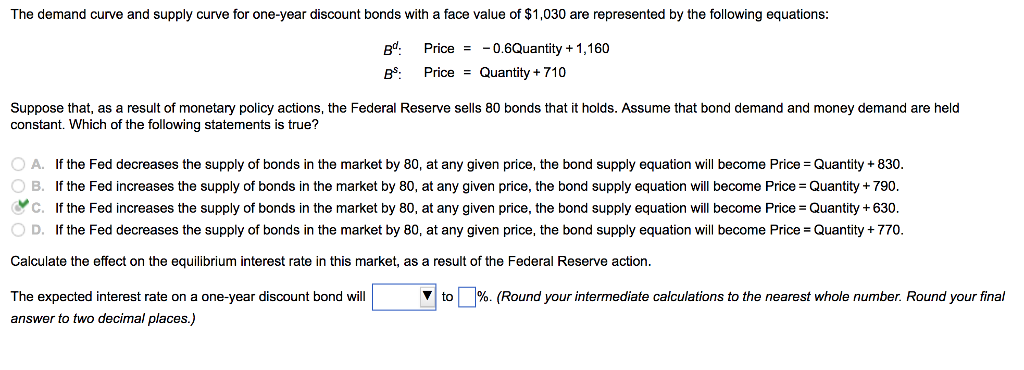I need help figuring out the second part of the question. The demand curve and supply curve for one-year discount bonds with a face value of \$1,030 are represented by the following equations gt. Price=-08Quantity +1,160 Suppose that, as a result of monetary policy actions, the Federal Reserve sells 80 bonds that it holds. Assume that bond demand and money demand are held constant. Which of the following statements is true? 0 A. O B. If the Fed decreases the...

• ### Suppose that you have been hired to analyze wages in a simple market. The demand for...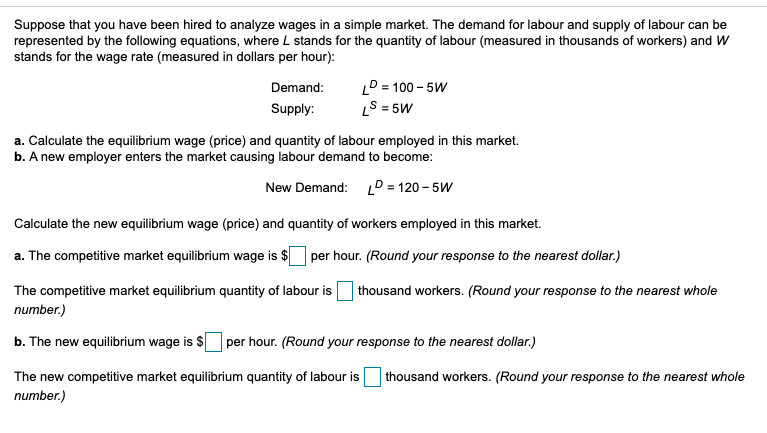Suppose that you have been hired to analyze wages in a simple market. The demand for labour and supply of labour can be represented by the following equations, where L stands for the quantity of labour (measured in thousands of workers) and W stands for the wage rate (measured in dollars per hour): Demand: Supply: P = 100 - 5W S = 5W a. Calculate the equilibrium wage (price) and quantity of labour employed in this market. b. A new...

• ### At \$0.34 per bushel, the daily supply for wheat is 401 bushels, and the daily demand...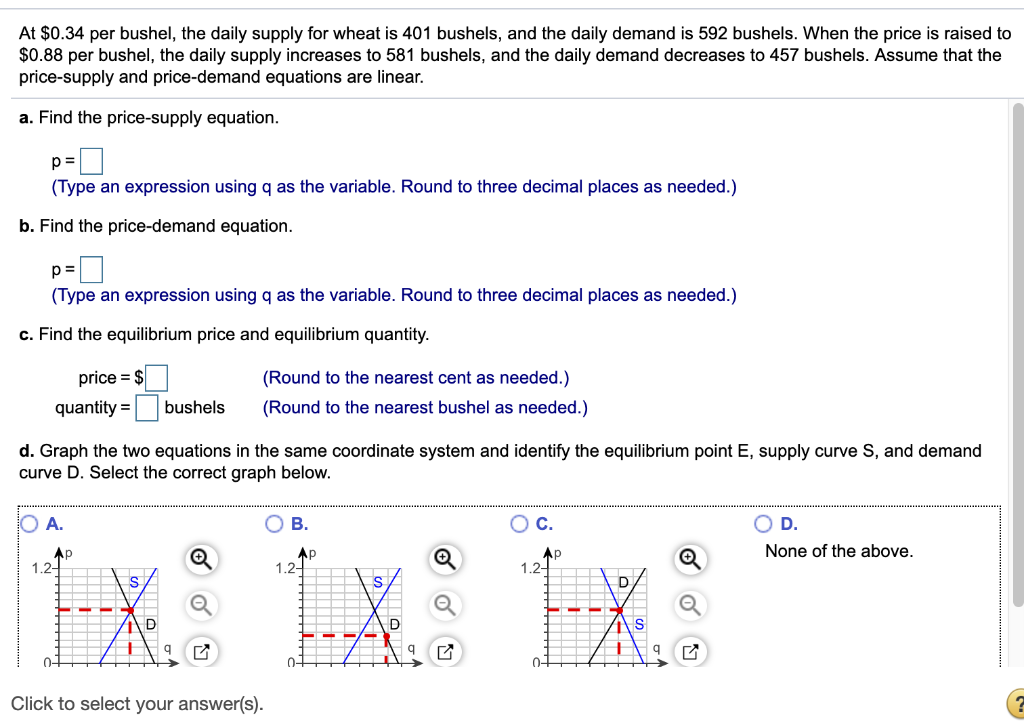At \$0.34 per bushel, the daily supply for wheat is 401 bushels, and the daily demand is 592 bushels. When the price is raised to \$0.88 per bushel, the daily supply increases to 581 bushels, and the daily demand decreases to 457 bushels. Assume that the price-supply and price-demand equations are linear. a. Find the price-supply equation (Type an expression using q as the variable. Round to three decimal places as needed.) b. Find the price-demand equation. (Type an expression...

• ### At \$0.36 per bushel, the daily supply for wheat is 372 bushels, and the daily demand...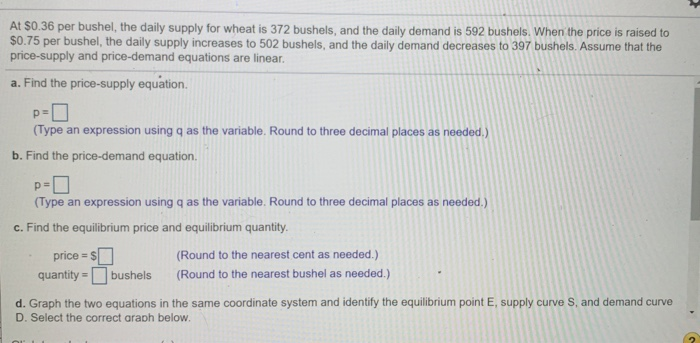At \$0.36 per bushel, the daily supply for wheat is 372 bushels, and the daily demand is 592 bushels. When the price is raised to \$0.75 per bushel, the daily supply increases to 502 bushels, and the daily demand decreases to 397 bushels. Assume that the price-supply and price-demand equations are linear. a. Find the price-supply equation. DE (Type an expression using q as the variable. Round to three decimal places as needed.) b. Find the price-demand equation. pa (Type...

• ### Suppose that the demand curve for wheat is Q=120 - 10p and the supply curve is...

Suppose that the demand curve for wheat is Q=120 - 10p and the supply curve is Q=10p The government imposes a price ceiling of p= \$4 per unit per unit. a. How do the equilibrium price and quantity​ change?  ​(round quantities to the nearest integer and round prices to the nearest​ penny) The equilibrium quantity without the price ceiling is 60 and the price without the price ceiling is ​\$6. The equilibrium quantity with the price ceiling is 40. B)...

• ### Suppose that the demand curve for wheat is Q=140 - 10p and the supply curve is...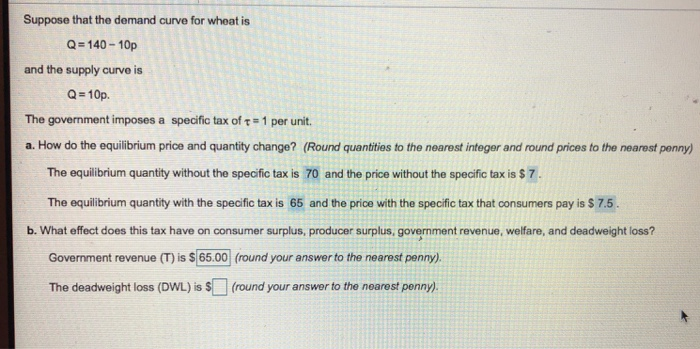Suppose that the demand curve for wheat is Q=140 - 10p and the supply curve is Q = 10p. The government imposes a specific tax of = 1 per unit. a. How do the equilibrium price and quantity change? (Round quantities to the nearest integer and round prices to the nearest penny) The equilibrium quantity without the specific tax is 70 and the price without the specific tax is \$ 7. The equilibrium quantity with the specific tax is 65...